# Splits and Networx

#### June 18, 2019

This tutorial gives a basic introduction for constructing phylogenetic networks and adding parameters to trees or networx objects using phangorn (Schliep 2011) in R. Splits graphs or phylogenetic networks are a useful way to display conflicting data or to summarize different trees. Here, we present two popular networks, consensus networks (Holland et al. 2004) and Neighbor-Net (Bryant and Moulton 2004).
Trees or networks are often missing either edge weights or edge support values. We show how to improve a tree/networx object by adding support values or estimating edge weights using non-negative Least-Squares (nnls).

We first load the phangorn package and a few data sets we use in this vignette.

library(phangorn)
data(Laurasiatherian)
data(yeast)

## consensusNet

A consensusNet (Holland et al. 2004) is a generalization of a consensus tree. Instead of only representing splits (taxon bipartitions) occurring in at least 50% of the trees in a bootstrap or MCMC sample one can use a lower threshold and explore competing splits. Note that, in its basic implementation used here, the consensusNet edge lengths are proportional to the frequence of the corresponding splits in the provided list of trees.

The input for consensusNet is a list of trees i.e. an object of class multiPhylo.

set.seed(1)
bs <- bootstrap.phyDat(yeast, FUN = function(x)nj(dist.hamming(x)),
bs=100)
tree <- nj(dist.hamming(yeast))
par("mar" = rep(1, 4))
tree <- plotBS(tree, bs, "phylogram")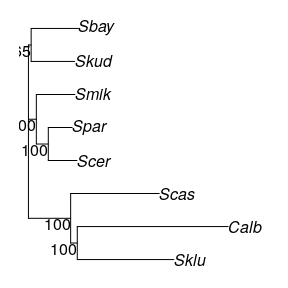cnet <- consensusNet(bs, .3)
plot(cnet, "2D", show.edge.label=TRUE)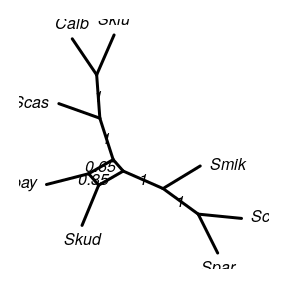In many cases, consensusNet will return more than two incompatible (competing) splits. This cannot be plotted as a planar (2-dimensional) graph. Such as situation requires a n-dimensional graph, where the maximum number of dimensions equals the maximum number of incompatible splits. For example, if we have three alternative incompatible splits: (A,B)|(C,D) vs. (A,C)|(B,D) vs. (A,D)|(B,C), we need a 3-dimensional graph to show all three alternatives. A nice way to get still a good impression of the network is to plot it in 3D.

plot(cnet)
# rotate 3d plot
play3d(spin3d(axis=c(0,1,0), rpm=6), duration=10)
# create animated gif file
movie3d(spin3d(axis=c(0,1,0), rpm=6), duration=10)

which will result in a spinning graph similar to this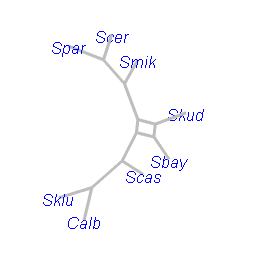rotatingNetworx

## neighborNet

The function neighborNet implements the popular method of Bryant and Moulton (2004). The Neighbor-Net algorithm is essentially a 2D-version of the Neighbor joining algorithm. The Neighbour-net is computed in two steps: the first computes a circular ordering of the taxa in the data set; the second step involves the estimation of edge weights using non-negative Least-Squares (nnls).

dm <- dist.hamming(yeast)
nnet <- neighborNet(dm)
par("mar" = rep(1, 4))
plot(nnet, "2D")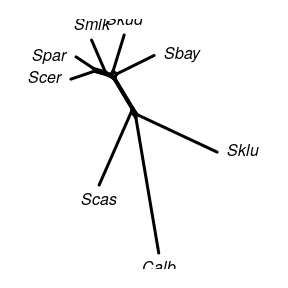The advantage of Neighbor-Net is that it returns a circular split system which can be always displayed in a planar (2D) graph. The plots displayed in phangorn may (however) not be planar, but re-plotting can give you a planar graph. This unwanted behavior will be improved in future version. The rendering of the networx is done using the the fantastic igraph package (Csardi and Nepusz 2006).

## Adding support values

We can use the generic function addConfidences to add (branch) support values from a tree, i.e. an object of class phylo to a networx, splits or phylo object. The Neighbor-Net object we computed above provides no support values. We can add the support values from the tree we computed to the splits (edges) shared by both objects.

nnet <- addConfidences(nnet, tree)
par("mar" = rep(1, 4))
plot(nnet, "2D", show.edge.label=TRUE)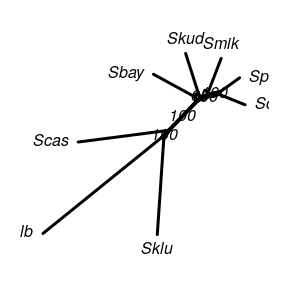Analogously, we can also add support values to a tree:

tree2 <- rNNI(tree, 2)
tree2 <- addConfidences(tree2, tree)
# several support values are missing
par("mar" = rep(1, 4))
plot(tree2, show.node.label=TRUE)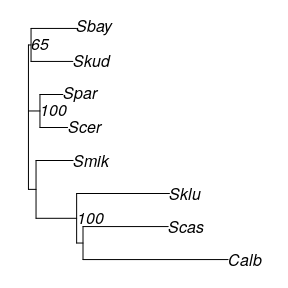## Estimating edge weights (nnls)

Consensus networks, on the other hand, provide primarily information about support values corresponding to a split, but no information about the actual difference between the taxon bipartitions defined by that split. For example, one may be interested how the alternative support values correspond with the actual genetic distance between the involved taxa. Given a distance matrix, we can estimate edge weights using non-negative Least-Squares, and plot them onto the consensusNet splits graph.

cnet <- nnls.networx(cnet, dm)
par("mar" = rep(1, 4))
plot(cnet, "2D", show.edge.label=TRUE)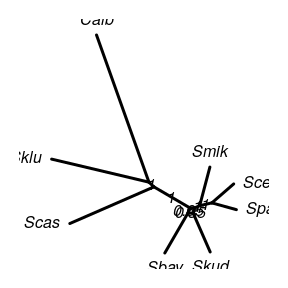### Import and export networks, advanced functions for networx objects

The functions read.nexus.networx and write.nexus.networx can read and write nexus files for or from SplitsTree (Huson and Bryant 2006). Check-out the new vignette IntertwiningTreesAndNetworks for additional functions, examples, and advanced applications.